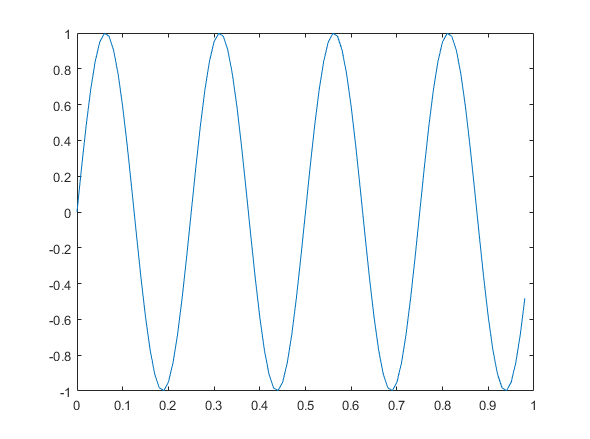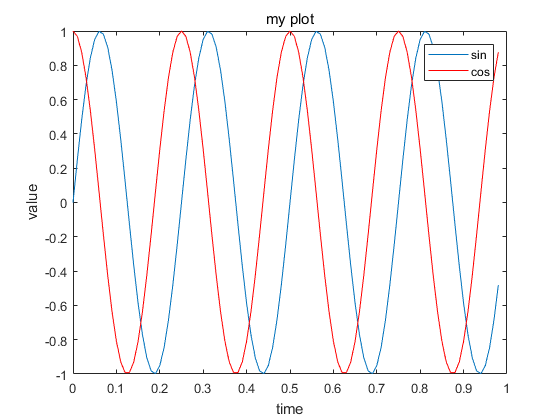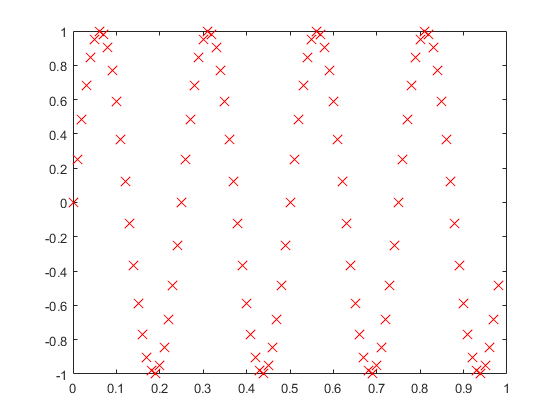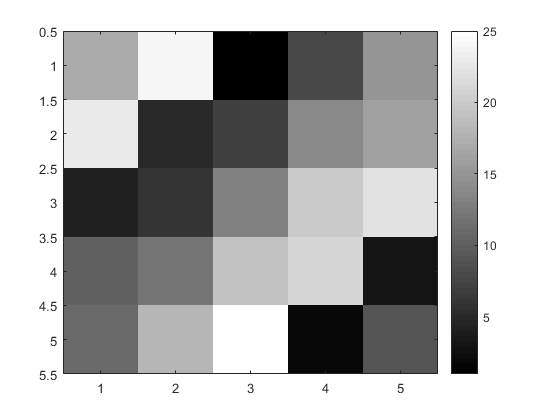• 简单介绍matlab中的常用命令 MATLAB命令大全 管理命令和函数 help 在线帮助文件 doc 装入超文本说明 what M、MAT、MEX文件的目录列表 type 列出M文件 lookfor 通过help条目搜索... Path 控制MATLAB的搜索路径
• MATLAB命令索引MATLAB命令索引管理命令和函数help在线帮助文件doc装入超文本说明whatM、MAT、MEX文件的目录列表type列出M文件lookfor通过help条目搜索关键字which定位函数和文件Demo运行演示程序Path控制MATLAB的...
MATLAB命令索引MATLAB命令索引管理命令和函数help在线帮助文件doc装入超文本说明what      M、MAT、MEX文件的目录列表type列出M文件lookfor通过help条目搜索关键字which定位函数和文件Demo运行演示程序Path控制MATLAB的搜索路径管理变量和工作空间Who列出当前变量Whos列出当前变量(长表)Load从磁盘文件中恢复变量Save保存工作空间变量Clear从内存中清除变量和函数Pack整理工作空间内存Size矩阵的尺寸Length向量的长度disp显示矩阵或与文件和*作系统有关的命令cd改变当前工作目录Dir目录列表Delete删除文件Getenv获取环境变量值!执行DOS*作系统命令Unix执行UNIX*作系统命令并返回结果Diary保存MATLAB任务控制命令窗口Cedit设置命令行编辑Clc清命令窗口Home光标置左上角Format设置输出格式Echo底稿文件内使用的回显命令more在命令窗口中控制分页输出启动和退出MATLABQuit退出MATLABStartup引用MATLAB时所执行的M文件Matlabrc主启动M文件一般信息Info      MATLAB系统信息及Mathworks公司信息Subscribe成为MATLAB的订购用户hostid      MATLAB主服务程序的识别代号
展开全文• 1.matlab中分割和拼接字符串的 save_path = 'H:\Experiment\Img\'; smap_list = 'P1_front_img.jpg'; cell_str = strsplit(smap_list,'_'); %分成三段： 'P1' 'front' 'img.jpg' smap_name = cell_str{1,1}; save_...
1.matlab中分割和拼接字符串的

save_path = 'H:\Experiment\Img\';
smap_list = 'P1_front_img.jpg';
cell_str = strsplit(smap_list,'_');  %分成三段： 'P1'    'front'    'img.jpg'
smap_name = cell_str{1,1};
save_full_path = strcat(save_path, smap_name, '.jpg');  % H:\Experiment\Img\P1.jpg

展开全文• 或者在命令窗口通过addpath命令添加路径，重启Matlab后，添加的路径没有保存。 原因：pathdef.m文件的权限问题 解决方法： cd /usr/local/MATLAB/R2012a/toolbox/local sudo chmod 777 pathdef.m
问题如下：在Matlab中通过File->Set Path...或者在命令窗口通过addpath命令添加路径，重启Matlab后，添加的路径没有保存。
原因：pathdef.m文件的权限问题
解决方法：
cd /usr/local/MATLAB/R2012a/toolbox/local
sudo chmod 777 pathdef.m

展开全文• disp(var) // display variable disp(sprintf(‘2 decimals: %0.2f’,a)) //format with two decimals format long // format short // ...pwd //reture the current path ls //display load
注：每条命令后面跟不跟分号的区别就是：不加分号执行命令后立即显示出来，加上分号不显示。  1.矩阵的建立

A = [1 2; 3 4; 5 6];  % 建立一个 3x2的矩阵、


>>  A = [1 2; 3 4; 5 6]

A =
1     2
3     4
5     6

v = [1 2 3]; %建立一个1x3的行向量

>> v = [1 2 3]

v =
1     2     3

v = [1;2;3]; %建立一个3维列向量

>> v = [1;2;3]

v =
1
2
3

v = 1:0.2:2;%建立一个行向量，从1开始，步长为0.2，到2结束

>> v = 1:0.2:2

v =
1.0000    1.2000    1.4000    1.6000    1.8000    2.0000

v = 1:6; %建立一个行向量，从1开始，步长为1，到6结束
v = 1:2:6; %建立一个行向量，从1开始，步长为2，到6结束  ones(2,3); %建立一个2x3的矩阵，元素全是1

>> ones(2,3)

ans =
1     1     1
1     1     1

zeros(2,3);%建立一个2x3的矩阵，元素全是0 c = 2*ones(2,3);%建立一个2x3的矩阵c，元素全是2

>> c = 2*ones(2,3)

c =
2     2     2
2     2     2

rand(2,3);%建立一个2x3的随机矩阵

>> rand(2,3)

ans =
0.8147    0.1270    0.6324
0.9058    0.9134    0.0975

randn(2,3); %同上
eye(4) % 4 x 4 单位矩阵

A = [1 2; 3 4; 5 6];  size(A); %返回A的行数和列数

>> A = [1 2; 3 4; 5 6];
>> size(A)
ans =

3     2

size(A,1); % 返回A的行数
size(A,2);% 返回A的列数
length(A);// 返回max(row,column)

2.数据操作

A = [1 2; 3 4; 5 6];

A =
1     2
3     4
5     6

A(3,2);% 得到第三行第二列的元素

>> A(3,2)

ans =
6

A(2,:); % 得到第二行的所有元素

>> A(2,:)

ans =
3     4

A(:,1) ;%得到第一列的所有元素。‘:’表示沿着每一行/列的所有元素

>> A(:,1)
ans =

1
3
5

A([1 3],:)%得到所有行号为1、3的元素，即第一行和第三行
A([1:2:7],:) %得到第一、三、五、七行

>> A([1 3],:)
ans

1     2
5     6

A(:,[1 2])%得到第一、二列

A(:,2) = [10;11;12] %用10,11,12来替换A的第二列

>> A(:,2) = [10;11;12]

A =
1    10
3    11
5    12

A(2,:) = [23 24] %用23 24来替换第二行

>> A(2,:) = [23 24]

A =
1    10
23    24
5    12

>> 

A = [A,[100;101;102]] %在A的右边加上一列

>> A = [A,[100;101;102]]

A =
1    10   100
23    24   101
5    12   102


注：如果若干元素在同一行，用逗号或空格隔开；若在同一列用分号隔开

A = [A;[7,8,9]] %在A的下方添加一行

>> A = [A;[7,8,9]]

A =
1    10   100
23    24   101
5    12   102
7     8     9


V=A(:) %让A的所有元素变成一列，并赋给V。


>> V = A(:)

V =

1
23
5
7
10
24
12
8
100
101
102
9

A = [1 2; 3 4]
B  = [6 7;8 9] C = [A B] ;%将A（左边） B（右边）组合成一个矩阵

>> C = [A B]

C =
1     2     6     7
3     4     8     9


C = [A;B]  %将A（上） B（下）组合成一个矩阵，使用的时候注意维度的匹配

>> C = [A;B]

C =

1     2
3     4
6     7
8     9


3.矩阵计算

M = [1 2;3 4;5 6] Y = [3 2] M - Y %M的第一列都减3，第二列都减2

>> M - Y

ans =
-2     0
0     2
2     4

M ./ Y %M的第一列都除以3，第二列都除以2

>> M ./ Y

ans =
0.3333    1.0000
1.0000    2.0000
1.6667    3.0000

M .* Y %M的第一列都乘以3，第二列都乘以2


>> M .* Y

ans =
3     4
9     8
15    12

A = [1 2; 3 4; 5 6]
B = [11 12; 13 14; 15 16]
C = [1 1; 2 2]  A*C % 3x2 * 2x2 = 3x2 的矩阵

>> A*C
ans =

5     5
11    11
17    17


A.*B % A和B的对应元素相乘（所有要就AB的维度要相同）

>> A.*B
ans =

11    24
39    56
75    96

A.^2 %得到A的每一个元素的平方

>> A.^2

ans =
1     4
9    16
25    36

A ./ 2 %A的每一个元素除以2

>> A ./ 2

ans =
0.5000    1.0000
1.5000    2.0000
2.5000    3.0000

2 ./ A %2除以A的每一个元素

>> 2 ./ A

ans =
2.0000    1.0000
0.6667    0.5000
0.4000    0.3333

log(A)
exp(A)
abs(A) %对A的每一个元素进行相应的运算

A’ %得到A的转置

>> A'

ans =
1     3     5
2     4     6

max(A) %得到每一列的最大元素，如果A是一个1xn的向量，则返回最大值

>> max(A)

ans =
5     6

max(max(A))%  返回的就是5，6中的最大值

>> max(max(A))

ans =
6

max(A,[],1) // 等同于 max(A) max(A,[],2) //得到每一行的最大值

>> max(A,[],2)

ans =
2
4
6

A < 3 %返回元素是否小于3的逻辑判断值

>> A < 3

ans =
3×2 logical 数组
1   1
0   0
0   0

find(A<3) %返回元素小于3的元素的编号

>> find(A<3)

ans =

1
4

[row,colunm] = find(A<3)%返回元素小于3的元素的行号和列号

>> [row,colunm] = find(A<3)

row =
1
1
colunm =
1
2
%即A中的（1，1），（1，2）都小于3

sum(A) %计算A中每一列的和，如果A为1xn的向量，则返回所有元素的和

>> sum(A)
ans =

9    12

sum(sum(A)) %返回9 12 的和，也就是A中所以元素的和，等同于sum(A(:))

>> sum(sum(A))
ans =

21

sum(A,1) %等同于 sum(A)，返回每一列的和 sum(A,2) %返回每一行的和

>> sum(A,2)

ans =
3
7
11

prod(A) %返回每一列各个元素的乘积，等同于prod(A,1)


>> prod(A)

ans =
15    48

prod(A,2) %返回每一行各个元素的乘积

**floor(r) %所有元素向下取整
ceil(r) % 所有元素向上取整**

>> r=rand(2)
r =
0.9649    0.9706
0.1576    0.9572

>> floor(r)

ans =

0     0

>> ceil(r)

ans =

1     1
1     1

flipud(eye(3))

>> flipud(eye(3))

ans =

0     0     1
0     1     0
1     0     0

pinv(A) %A的逆运算

>> pinv(A)

ans =

-1.3333   -0.3333    0.6667
1.0833    0.3333   -0.4167

4.作图

t = [0:0.01:0.98]
y1 = sin(2*pi*4*t) plot(t,y1)y2=cos(2*pi*4*t);
hold on; %表示画下一个图的时候，上一个图不消失，可以使两幅图在一个窗口中
plot(t,y2,’r’)%’r’ 表示曲线颜色
xlabel(‘time’)
ylabel(‘value’) %添加横纵坐标标签
legend(‘sin’,’cos’) %标识曲线
title(‘my plot’)%添加名称plot(t,y1,’rx’,’MarkerSize’,10) % ‘rx’ 代表用红叉作图，大小为10print -dpng ‘myPlot’ %导出图片到当前路径

figure(1); plot(t,y1);%打开窗口一，并画图（可以两个命令同时执行，用分号隔开）
figure(2); plot(t,y2); %打开另外一个窗口二，作图

subplot(1,2,1) // divides plot in a one-by-two grid, access first element
subplot(1,2,2)
plot(t,y2,’r’)
axis([0.5 1 -1 1])%设置X的范围
clf //clear figure

imagesc(A) //视觉化矩阵A
imagesc(magic(5)), colorbar, colormap gray %用灰度图视觉化5.其他

mean(M,1) % 计算每一列的平均值
mean(M,2) %  计算每一行的平均值
std(M) % 计算每一列的标准差，等同于(M,0,1)
std(M,0,2) % 计算每一行的平均值
size(M,1) % 一共多少行
size(M,2) % 一共多少列
numel(A) %返回一共有多少个元素
plot(J,’-b’,’Linewidth’,2) % plot a line in blue with width equal to 2
plot(X,’rx’,’MarkerSize’,10,’LineWidth’,20) % plot figure with red cross and width equal to 20

disp(var) // display variable
disp(sprintf(‘2 decimals: %0.2f’,a)) //format with two decimals
format long //
format short //
hist(w) //画出w值的直方分布图
pwd //reture the current path
ls //display
load filename //loading file
load(‘filename’) //loading file
who //show the all varable in current memory
whos //show the all varable of detailed view
// save and load data
load priceY //load a data set (a vector) named priceY
v = priceY(1:10)// v will be a vector with first 10 elements in priceY

load dataX //load a data set (a matrix) named dataX
v = dataX(1:10) // v will be a 1 by 10 matrix with first 10 elements in first column of dataX
save hello.mat v; // save the variable V into a file called hello.mat at current direction
clear // deletest all of the variables in your workspace 
展开全文• MATLAB常用命令速查（一） 1．管理命令和函数 addpath 添加目录到MATLAB搜索路径 doc 在Web浏览器上现实HTML文档 help 显示Matlab命令和M文件的在线帮助 helpwin helpdesk help lookfor 在基于Matlab搜索...
• 管理命令和函数addpath添加目录到MATLAB搜索路径doc在Web浏览器上现实HTML文档help显示Matlab命令和M文件的在线帮助helpwinhelpdesk helplookfor在基于Matlab搜索路径的所有M文件中搜索关键字partialpath 部分路径名...
• MATLAB命令大全 MATLAB命令大全 管理命令和函数 help 在线帮助文件 doc 装入超文本说明 what M、MAT、MEX文件的目录列表 type 列出M文件 lookfor 通过help条目搜索关键字 ... Path 控制MATLAB的搜索路径
• DIR List directory.  DIR directory_name lists the files in a directory. Pathnames and  wildcards may be used. For example, DIR *.m lists all the M-files  in the current directory.    ...
• fid=fopen('path','r'); while(~feof(fid))  info=fgets(fid);%按行读取  index=strfind(info,'a');%字符串查找  bbox=str2num(info);%字符串、数字的相互转化 end 写txt： fid=fopen('path','w'); fprintf(fid,'...
• matlab中常用到文件操作命令，列表如下，如果后续用到，可在matlab help中详细查询使用方法。1. dir %列出文件夹内容2. cd path %由当前目录进入path目录举例： cd E:\matlab3. movefile %剪切文件4. copyfile % ...
• 1. exist - exist file or other things if~exist(metaDir, 'file');  metaDir = '\\netapp-ma\public\stereo_of_data\';...2. fullfile - complete the file path dataDir= fullfile(metaDir, '4
• 管理命令和函数 help 在线帮助文件 doc 装入超文本说明 what M MA TMEX 文件的目录列表 type 列出 M 文件 lookfor 通过 help 条目搜索关键字 which 定位函数和文件 Demo 运行演示程序 Path 控制 MATLAB 的搜索路径 ...
• ## MATLAB常用命令大全

千次阅读 2009-11-25 14:10:00
转自：http://tieba.baidu.com/f?kz=239279502 管理命令和函数 help 在线帮助文件 doc 装入超文本说明 what M、MAT、MEX文件的目录...Demo 运行演示程序 Path 控制MATLAB的搜索路径 管理变量和工作空间 W算法 hex 网格
• Path 控制MATLAB搜索路径 管理变量和工作空间 Who 列出当前变量 Whos 列出当前变量(长表) Load 从磁盘文件中恢复变量 Save 保存工作空间变量 Clear 从内存中清除变量和 Pack 整理工作空间内存 Size 矩阵尺寸 Length ...
• 以下只是函数的基本使用例子，详情使用help或者doc查看 ...imread('/path/filename'); // f =imread(/path/name.imageformat ); 二维矩阵f保存 图像输出 imwrite('/path/filename' ); // 图像显示 imshow(f)图像处理
• matlab开发-searchPath。除了在系统路径中搜索可执行文件外，此命令的工作方式与哪个命令类似。数学
• path：控制matlab的目录搜索路径 addpath：将目录添加到matlab的搜索路径上 rmpath：从matlab的搜索路径上删除目录 whatsnew：显示matlab的工具箱和readme文件 what：列出相应目录下的M文件、MAT文件和ME...
• MATLAB命令大全.doc MATLAB命令大全 管理命令和函数 help 在线帮助文件 doc 装入超文本说明 what M、MAT、MEX文件的目录列表 type 列出M文件 ... Path 控制MATLAB的搜索路径 管理变量和工作空间
• MATLAB命令大全管理命令和函数 help 在线帮助文件 doc 装入超文本说明 what M、MAT、MEX文件的目录列表 type 列出M文件 lookfor 通过help条目搜索关键字 which 定位函数和文件 Demo 运行演示程序 ...
• 管理命令和函数 help 在线帮助文件 doc 装入超文本说明 what M MAT MEX 文件的目录列表 type 列出 M 文件 lookfor 通过 help 条目搜索关键字 which 定位函数和文件 Demo 运行演示程序 Path 控制 MATLAB 的搜索路径 ...
• 记在这里，关于【pathMATLAB各种字符简单语句
• MATLAB 命令大全管理命令和函数help 在线帮助文件doc 装入超文本说明what M、MAT、MEX 文件的目录列表type 列出M 文件lookfor 通过help 条目搜索关键字which 定位函数和文件Demo 运行演示程序Path 控制MATLAB 的搜索...算法 hex 网格...

# matlabpath命令matlab 订阅